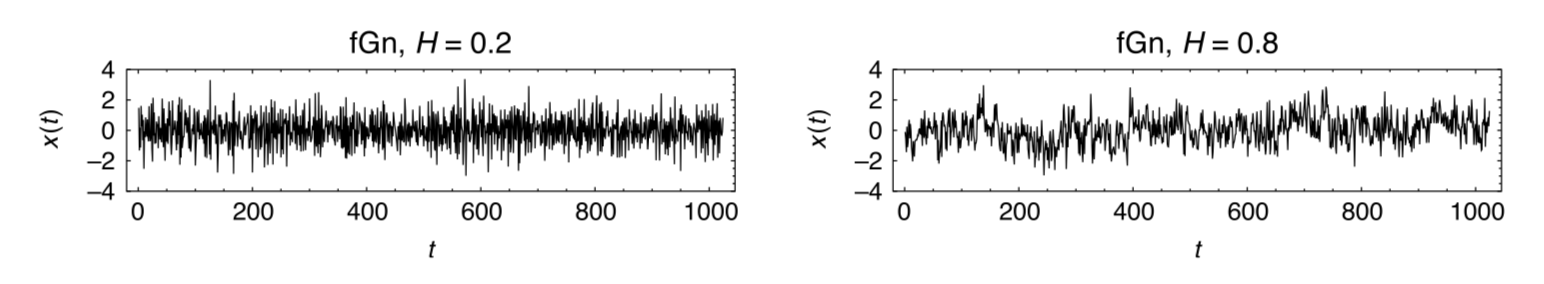# Are Holder Condition and signal to noise ratio (SNR) related?

This question was posted in https://math.stackexchange.com but I got hardly any view. If posting here is an objection please let me know I would delete it immediately.

This question has evolved from watching the video: https://www.youtube.com/watch?v=dh5hHpJ79jc

In the video the speaker talked about white noise case and $\alpha$ related to the Holder condition, (wikipedia link to Holder condition https://en.wikipedia.org/wiki/H%C3%B6lder_condition)

The question: Is there a relation between SNR (https://en.wikipedia.org/wiki/Signal-to-noise_ratio) and Holder condition?

Let me be more specific, As I understand, Rough path theory (https://en.wikipedia.org/wiki/Rough_path) is related to the solution of differential equation driven by non-smooth signals and non-smoothness is defined in terms of Holder condition. In the video it clearly relates the white noise case to the value of $\alpha$. Consider our driver is simple sine wave, which is very smooth, but if we add a noise then we can define SNR of the driver based on the strength of the noise and at the same time can we define $\alpha$ based on the strength on the noise?

In some sense I am trying to define rough path theory in terms of SNR, is it possible? Any comments would be highly appreciated

• Hölder exponent $h$, Hurst exponent $H$, and fractal dimension $D$ (with $D=2-H=1-h$) describe the correlations in the fluctuations of the signal, not the strength of the fluctuations as quantified by the SNR. – Carlo Beenakker Jul 10 '18 at 8:16
• @CarloBeenakker Thank you for your comments, but I did not understand how it is relates to the correlations. Mmay I request few more explanation or references which would help me to understand. – Creator Jul 10 '18 at 8:32

The Hölder exponent $h$ and the Hurst exponent $H$ (related by $h=H-1$ for a continuous signal) characterise correlations in the noise, not the size of the noise (as quantified by the signal-to-noise ratio). So these are different quantities. This plot illustrates two signals with the same signal-to-noise ratio but different $H$ (short-term correlations on the left, long-term correlations on the right).source: On the relationship between the Hurst exponent, the ratio of the mean square successive difference to the variance, and the number of turning points
• just visual inspection: the curve $x(t)$ has variance close to unity in both plots. – Carlo Beenakker Jul 19 '18 at 6:29